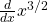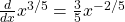# 3.2: Power Rule

$$\newcommand{\vecs}{\overset { \rightharpoonup} {\mathbf{#1}} }$$ $$\newcommand{\vecd}{\overset{-\!-\!\rightharpoonup}{\vphantom{a}\smash {#1}}}$$$$\newcommand{\id}{\mathrm{id}}$$ $$\newcommand{\Span}{\mathrm{span}}$$ $$\newcommand{\kernel}{\mathrm{null}\,}$$ $$\newcommand{\range}{\mathrm{range}\,}$$ $$\newcommand{\RealPart}{\mathrm{Re}}$$ $$\newcommand{\ImaginaryPart}{\mathrm{Im}}$$ $$\newcommand{\Argument}{\mathrm{Arg}}$$ $$\newcommand{\norm}{\| #1 \|}$$ $$\newcommand{\inner}{\langle #1, #2 \rangle}$$ $$\newcommand{\Span}{\mathrm{span}}$$ $$\newcommand{\id}{\mathrm{id}}$$ $$\newcommand{\Span}{\mathrm{span}}$$ $$\newcommand{\kernel}{\mathrm{null}\,}$$ $$\newcommand{\range}{\mathrm{range}\,}$$ $$\newcommand{\RealPart}{\mathrm{Re}}$$ $$\newcommand{\ImaginaryPart}{\mathrm{Im}}$$ $$\newcommand{\Argument}{\mathrm{Arg}}$$ $$\newcommand{\norm}{\| #1 \|}$$ $$\newcommand{\inner}{\langle #1, #2 \rangle}$$ $$\newcommand{\Span}{\mathrm{span}}$$$$\newcommand{\AA}{\unicode[.8,0]{x212B}}$$

“I’ve got the power!” –Snap (German band)

If you apply the definition of the derivative to several functions, you’ll see:

$$\begin{array}{cc} \frac{d}{dx} x^0 & 0 \\ \frac{d}{dx} x^1 & 1\\ \frac{d}{dx} x^2 & 2x \\ \frac{d}{dx} x^3 & 3x^2 \\ \frac{d}{dx} x^4 & 4x^3 \end{array}$$

See the pattern?

$$\boxed{\frac{d}{dx} x^n = n x^{n-1}}$$

As we’ll see in the next section, this even works for non-integers. The key is to multiply by the exponent, then decrease the exponent by one.

The next rules say that constant multiples and addition work nicely.

$$\boxed{\frac{d}{dx} c \cdot f(x) = c f'(x)}$$

$$\boxed{\frac{d}{dx} [f(x) + g(x)] = f'(x) + g'(x)}$$

The key is you can just worry about the derivative of each piece of a sum separately. Constant multiples “come along for the ride”. With only these three rules, you can now take the derivative of any polynomial. Check it out.

##### Power

Find the following derivatives.

1. $$\frac{d}{dx} 3x^2$$

To do this one, we use the power rule on the $$x^2$$ part, and get $$2x$$. However, we are also multiplying by $$3$$, so the answer is multiplied by $$3$$ as well. Hence the answer is $$3(2x) = \boxed{6x}$$.

2. $$\frac{d}{dx} x^3 + x$$

By the power rule, we find $$\frac{d}{dx} x^3 = 3x^2$$, and $$\frac{d}{dx} x$$ is $$\frac{d}{dx} x^1$$ which becomes $$1x^0$$ by the power rule, which is $$1$$. By the addition rule, we have $$\frac{d}{dx} x^3 + x = \boxed{3x^2 + 1}$$.

3. $$\frac{d}{dx} 2x^3 + 5$$

You take the derivative of $$x^3$$ and you have $$3x^2$$. Times by $$2$$, that leaves $$6x^2$$. Okay, about the five? It is tempting to leave the five put, but actually $$\frac{d}{dx} 5 = 0$$. Why? Well, it’s a constant, so it does not affect the slope. Hence we get $$\boxed{6x^2}$$.

## More power rule examples

Note that the power rule works with fractional and negative exponents as well! Here are some examples.

##### Fractional

Find.

To apply the power rule in this case, we need to first multiply by the exponent (), then subtract one from the exponent. Then we have##### Negative

Find $$\frac{d}{dx} 7x^{-2}$$.

In this problem, we just worry about the $$x^{-2}$$ to start with. We multiply by the exponent $$({\color{red} -2})$$, and then subtract one from that to get $${\color{blue} -2 - 1 = -3}$$. Then we have

$$\frac{d}{dx} 7x^{-2} = 7 ({\color{red} -2} x^ ParseError: invalid DekiScript (click for details) Callstack: at (Bookshelves/Calculus/Informal_Calculus_with_Applications_to_Biological_and_Environmental_Sciences_(Seacrest)/03:_Rules_for_Derivatives/3.02:_Power_Rule), /content/body/div/div/div/p/span, line 1, column 1  ) = \boxed{-14 x^{-3}}$$

Sometimes there are hidden fractional or negative exponents. Don’t let them fool you, they are just like the examples above. Just remember these rules:

$$\boxed{\frac{1}{x^n} = x^{-n}}$$Let’s see some examples.

##### Roots

Find

1. $$\frac{d}{dx} \sqrt{x}$$

This problem is much easier if we can rewrite the $$\sqrt{x}$$. This is the same thing as, and hence we have2. $$\frac{d}{dx} 5\sqrt{x}$$

To solve this, it really helps to rewrite $$\sqrt{x}$$ as. Once you do that, this prolem is just the power rule and constant multiple rule:3. $$\frac{d}{dx} \sqrt{x^3} + x^2 + 7$$

Focus on the easy parts first: we know $$\frac{d}{dx} x^2 = 2x$$, and we know that $$\frac{d}{dx} 7 = 0$$. So we just need to figure out the $$\frac{d}{dx} \sqrt{x^3}$$. What is this? Well, we can rewrite this as. So now it is just the power rule, and we multiply byand subtract to get. Hence. Putting it all together:##### Powers of $$x$$ in the denominator

1. $$\frac{d}{dx} \frac{1}{x}$$

We can rewrite $$\frac{d}{dx} \frac{1}{x} = \frac{d}{dx} \frac{1}{x^1}$$ as $$\frac{d}{dx} x^{-1}$$. Now we apply the power rule:

$$\frac{d}{dx} \frac{1}{x} = \frac{d}{dx} x^{-1} = \boxed{-1 x^{-2}}.$$

2. $$\frac{d}{dx} \frac{4}{x^2}$$

We can think of $$4$$ as just a constant out front, and hence we want $$\frac{d}{dx} 4 \left( \frac{1}{x^2} \right )$$. We can then rewrite $$\frac{1}{x^2}$$ as $$x^{-2}$$. And we want $$\frac{d}{dx} 4(x^{-2})$$. Using the power rule, we multiply by $$-2$$ and subtract one, and we have

$$\frac{d}{dx} \frac{4}{x^2} = \frac{d}{dx} 4x^{-2} = \boxed{-8 x^{-3}}.$$

3. $$\frac{d}{dx} \frac{1}{\sqrt{x}}$$

This combines the fractional and denominator stuff. We first rewrite $$\sqrt{x}$$ as:We then rewrite as a negative fractional exponent.Finally, we use the power rule.This page titled 3.2: Power Rule is shared under a CC BY 4.0 license and was authored, remixed, and/or curated by Tyler Seacrest via source content that was edited to the style and standards of the LibreTexts platform; a detailed edit history is available upon request.